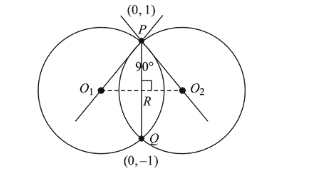# Two circles with equal radii are intersecting at the pointsQuestion:

Two circles with equal radii are intersecting at the points $(0$, 1) and $(0,-1)$. The tangent at the point $(0,1)$ to one of the circles passes through the centre of the other circle. Then the distance between the centres of these circles is :

1. (1) 1

2. (2) 2

3. (3) $2 \sqrt{2}$

4. (4) $\sqrt{2}$

Correct Option: , 2

Solution:

$\because$ Two circles of equal radii intersect each other

orthogonally. Then $R$ is mid point of $P Q$.and $P R=O_{1} R=O_{2} R$

$P R=\frac{1}{2} \sqrt{(0-0)^{2}+(1+1)^{2}}=1$

$\therefore$ Distance between centres $=1+1=2$.